# 3. Basic Theory

## 3. Basic Theory

This section discusses theories related to this research. The section first presents a video model built on the content feature space. This is followed by a discussion of the feature spaces that were adopted in the research. Next, the observations on video transitions and the modeling of video transitions are discussed in a multi-temporal-resolution manner. Lastly, wavelets to model the multi-temporal resolution phenomenon are introduced.

### 3.1 Video Model

Video can be mathematically modelled through the content features of the frames in the video data stream. The content of the frames is normally represented by some low-level features such as the color, shape, texture or motion. By considering a specific feature such as the color, a video stream can be represented as a trajectory in a multi-dimensional space, in this case, color space.

Figure 8.3 illustrates the trajectory of a video stream in a RGB 3-D color space. For illustration purposes a very simple mapping is performed, by computing the average RGB color for every frame in the video stream using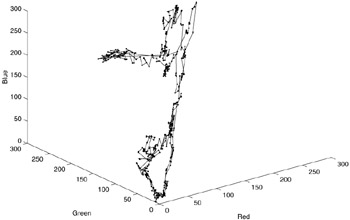Figure 8.3: Transitions on the video trajectory in RGB color space.

 (8.1)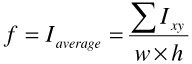where Ixy = (Rxy, Gxy, Bxy) represents the RGB value of every pixel (x, y) of the frame, and w and h are respectively the width and height of the frame. In other words, only one average color is used to represent a frame. In this way, every frame is mapped into a point in the RGB color space and connecting the points in their temporal order of occurrence in the video stream will map the temporal sequence of frames into a spatial trajectory of points in the color space. As a result, every video can be mapped into a spatial trajectory in a preferred feature space.

Different content features extracted from a video stream will lead to different feature spaces where the video will be mapped in a different corresponding trajectory. The dimension of a feature space depends on the dimensionality of the chosen feature. In the illustration of Figure 8.3, RGB forms a 3-D feature space. If a 64-bin color histogram is used to represent each frame, a 64-D feature space will be obtained. If the feature space is well chosen and corresponds closely by the user's perception of video contents, the similarity between any two frames is expected to be modelled closely by the distance between the two corresponding points in the space. Thus, similar frames will be closer in the feature space and dissimilar frames will be farther apart.

Assume that n -color histogram is used to represent the contents of each frame in a video. Every frame in the video can be represented as a frame vector

 (8.2)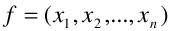where xi is the ith bin of the color histogram. Then, the temporal video stream can be shown as a feature vector stream

 (8.3)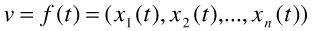### 3.2 Feature Space

To map the video into a trajectory in the feature space, feature descriptions of every frame must be obtained. In the uncompressed video stream where the frames are already obtained after decompression, the feature descriptions can be calculated by using image processing and machine vision techniques. For example, a 64-bin histogram description for the frame is a good feature description in color space and it can be obtained by first quantizing the image into 64 colors and then counting the pixels for every color.

In the compressed domain, image feature description of every frame is not obtained so easily as in uncompressed domain. This is because the data in the compressed video stream are DCT transformed, and the compressed video stream has its special structure that needs special treatment. To extract the image feature description, it is usually necessary to analyze the DCT coefficients for every frame. For each 8x8 blocks of a single DCT-based encoded video frame f, the DC coefficient gives the average luminance value of the whole block. In this case, all the DC coefficients for the whole frame can already be used to construct a meaningful image description about this frame at a lower spatial resolution.

While the DC coefficients can be obtained directly for I frames, it is necessary to apply motion compensation in order to extract them from the B or P frames. This is because in the MPEG coding system, the B or P frames only record the difference from reference frames for better efficiency. A method of extracting DC values from compressed MPEG stream is discussed in .

Thus, the image descriptions of every frame of the video stream can be obtained in both the uncompressed and compressed domains. Using the image descriptions, the video stream is mapped into the trajectory in the feature space. The mapping is actually a dimensionality reduction process. Usually, the video data is presented in a high dimensionality vector of the form

 (8.4)where In is the nth frame image description, x and y are the pixel location in the image, I(x, y) is the color of the pixel location at (x, y) and w and h are the width and height of the image. The feature description of the video frame is

 (8.5)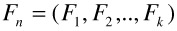where Fn is the n th frame feature description, and k is the dimension of the feature space.

In most cases, k is much smaller than w h. For an example, using a 64-bin histogram to represent a 320x240 resolution video frame reduces the dimensionality of the frame by 1/1200. The feature space must be chosen such that it is able to effectively discriminate frames. Thus, it is very important to choose a well-defined feature space.

Besides having a low dimension, other desirable properties of a feature space for video segmentation are as follows:

• It must have a reliable similarity measure and must model the user's perception of video sequence well.

• It must have a fixed dimensionality to form a stable feature space.

• It must be easy to extract the feature vector "automatically" from the video stream.

###### Feature Space for Global Feature -Histogram Representations

In the raw domain of video where frames are processed after full decompression, color histogram is the most basic and effective representation of image content of every frame. It models the global content of the image and provides a good basis for evaluating different images. Even in the compressed domain, by extracting the information from the DC coefficients, a DC histogram for every frame can also be obtained.

In this research, histogram-based methods for both the uncompressed (to form the 64-bin histogram) and the compressed video streams (to form the 64-bin DC histogram) have been implemented. The cost of computing the histogram is very low. For a good trade-off between efficiency and the quality of representation, 64-bin color histogram has been shown experimentally to be sufficient for most video processing applications, including video segmentation.

###### Feature Space for Region-Based Feature -DC64

Since the DC coefficients for every frame can be extracted and directly reflect the average luminance value of the block, the image description formed by DC coefficients already formed a good basis for a region-based representation of every frame. The feature vector for every frame is

 (8.6)where dc's are the DC coefficients for every block. This description can easily be used to extract the color distribution information of a frame with a 1/64-dimensionality reduction. This is called the DC image of the current frame. To achieve even lower dimensionality, the frame can be equally split into 8 by 8 sub-windows and the DC coefficients are averaged in the sub-window. Composing these 64 values can form a reduced DC image that is 8 by 8 in size. This DC64 image, or the reduced DC image, represents the spatial information that can be used as basis to represent the color-spatial information of the frame.

###### Feature Space for Motion-Based Feature - MA64

Since the motion vectors and the macroblock type information can be easily extracted from the MPEG compressed stream, it forms a good basis for a motion-based representation of every frame. The major problem in shot boundary detection is the flash and camera/object motion resulting in wrong transition detection. The motion based feature vector is robust in characterizing motion accurately and is used to identify: a) transition boundary points; and b) elimination of wrong transitions due to motion. A motion angle (MA) histogram representing the directions of motion is constructed. The motion vector direction is quantized to 60 values with each bin representing 6-degree increment from 0 to 360 degrees. This feature vector is concatenated with 4 more values representing counts of forward, backward, intra and skipped macroblocks in the frame. This forms the compact representation of a MA64 feature vector.

 (8.7)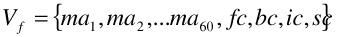where ma's are the motion angles, fc is the forward predicted motion vector count, bc is the backward predicted motion vector count, ic is the intra-coded macroblock count and sc is the skipped macroblock count. This feature vector characterizes motion continuity and is used to observe the level of motion activity in the video sequence.

### 3.3 Multi-Resolution Phenomena of Transitions

One of the advantages of modeling video as a trajectory in the feature space is that it provides a natural visual representation of video stream that can be observed easily. Similar frames are closer on the trajectory in the space and different frames are farther away from each other. Thus, video transitions can be studied by observing the video trajectory in the feature space.

It is easily shown from Figure 8.3 that video shot transitions correspond to regions where the contents change rapidly. These changes represent the discontinuities in the trajectory and can be considered as the boundaries in a video, similar to the concept of edges in images. Thus, algorithms similar to edge detection in computer vision can be applied since all these points of rapid variations can be identified as local maxima in the first order derivatives of the video signals in a suitable domain.

By empirically observing GTs in many video streams, it is found that different types of GTs exist like fade-in/fade-out, dissolve, wipe and morphs. Moreover, the length of the transitions can vary widely. Sample MPEG-7 data shows that GT length can vary from 2 frames to several hundreds frames (e.g., 487 frames). In this case, it cannot be claimed that all the video transitions will bring in sufficiently "rapid" changes in the video frame content (or in the video trajectory in a proper feature space). Instead, it is true that whatever is the type or the length of the transition there will always be a change big enough that is observable at some temporal resolution. So, the transitions must be defined with respect to different resolutions. By viewing the video at multiple resolutions simultaneously, the detection of both CUTs and GTs can be unified. By employing a framework of temporal multi-resolution analysis, the information across multiple temporal resolutions can also be utilized to solve the problem of video segmentation.

### 3.4 Wavelets

Based on the observations stated in the previous section, video transitions can be uniformly modeled as maxima of first order derivatives in some resolutions of the video signal. Thus, wavelets can be applied in solving the problem of video segmentation. [20

Consider the temporal sequence of video frames as samples from a continuous signal f(t), and the video signal in the ath temporal resolution is denotated as fa (t). Denoting the Gaussian kernel by

 (8.8)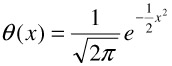then fa(t) can be obtained by convolving f(t) with θ(t)

 (8.9)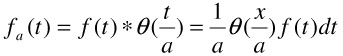Here, the temporal resolution means sampling video stream by grouping frames. The higher is the a, the lower the temporal resolution will be, and the coarser the sampling will become. This is because the convolution of f(t) and θ(t) removes the details (high frequency components) of f(t) in time and spatial domain. Since both CUT and GT all correspond to the maxima of the first order derivatives in low resolution video signal, these transitions can be detected by simply calculating the first order derivative of fa(t) when a is sufficiently large.

The whole process of computing fa(t) in different resolutions and calculating the first order derivative is equivalent to applying Canny wavelets transformation on f(t). Canny wavelets are defined from the first order derivative of the Gaussian kernel. The reason for n derivatives of the Gaussian θ

 (8.10)to be wavelets for n > 0 can be shown by the lemma that follows:

Definition: Let n N. A wavelet Ψ has n vanishing moments (i.e., of order n), if for all integers k < n:

 (8.11)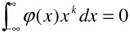and

 (8.12)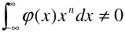Lemma: Let φ be an n-times differentiable function and φ(n) L2(R),φ(n) 0. Then, it follows that Ψ = φ(k) is a wavelet.

Denoting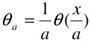as the Gaussian at resolution a, then the following can be obtained:

 (8.13)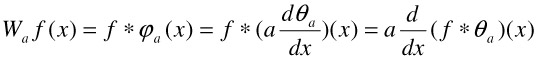Canny wavelets, which are shown in Figure 8.4, are derived from the first order derivative of the Gaussian kernel as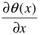. Here, it shows that the wavelet transform using Canny wavelets will obtain the first order derivative of the f(t) at various resolutions. Thus, the problem of video transition detection can be simplified to local maxima detection after wavelet transformation.Figure 8.4: Canny wavelets in RO-R3.

Figure 8.5 shows the result of using Canny wavelet transformation on a daily video stream in different temporal resolution. By carefully examining the maxima corresponding to video transitions, it can be noticed that a GT, which is not observable at a high resolution (scale 0), can be easily detected at a lower resolution (scale 3).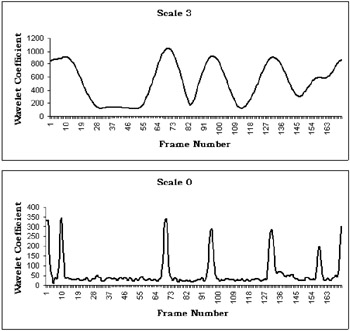Figure 8.5: Transitions shown at two resolutions.

Although all the transitions are shown in low resolution, different phenomena can be observed at different resolutions. For CUT, the significant maxima are observable both at a high resolution and at a coarser resolution. On the other hand, GTs only show the maxima at coarse temporal resolutions. By using wavelet transform, the analysis of the information across resolutions becomes easy.

Beside the convenience of the correspondence of maxima and the boundary, wavelet also provides a simple way to measure the regularity of the signal by using Lipschitz exponent α. It is proved that

 (8.14)The scale space can be constructed by dyadic scale 2j. Thus, the evolution of the amplitudes of the absolute maxima can be traced across the scales. Then, the degree of smoothness and the corresponding scale where the transition exists can be obtained. Using this information, it is possible to distinguish the CUT's from GT's.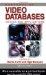Handbook of Video Databases: Design and Applications (Internet and Communications)
ISBN: 084937006X
EAN: 2147483647
Year: 2003
Pages: 393